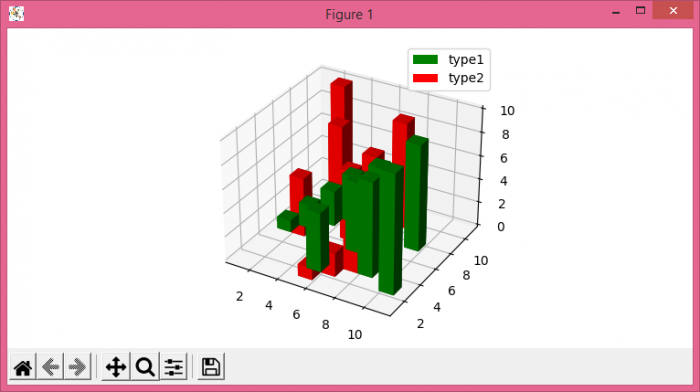# How to create a legend for a 3D bar in Matplotlib?

To create a legend for a 3D bar in matplotlib, we can plot 3D bars and place a legend using legend() method.

## Steps

• Set the figure size and adjust the padding between and around the subplots.
• Create a new figure or activate an esxisting figure using figure() method.
• Add an axes to the figure as part of a subplot arrangement.
• Create a list of data x3, y3, z3, dx, dy and dz using numpy.
• Plot a 3D bar using bar3d() method.
• Create a rectangle axis for legend placement.
• Use legend() method to place the legend for bars.
• To display the figure, use show() method.

## Example

import numpy as np
from matplotlib import pyplot as plt
plt.rcParams["figure.figsize"] = [7.50, 3.50]
plt.rcParams["figure.autolayout"] = True
fig = plt.figure()
x3 = [1, 2, 3, 4, 5, 6, 7, 8, 9, 10]
y3 = [5, 6, 7, 8, 2, 5, 6, 3, 7, 2]
z3 = np.zeros(10)
dx = np.ones(10)
dy = np.ones(10)
dz = [1, 2, 3, 4, 5, 6, 7, 8, 9, 10]
ax1.bar3d(x3, y3, z3, dx, dy, dz, color="green")
b1 = plt.Rectangle((0, 0), 1, 1, fc="green")
ax1.bar3d(y3, x3, z3, dx, dy, dz, color="red")
b2 = plt.Rectangle((0, 0), 1, 1, fc="red")
ax1.legend([b1, b2], ['type1', 'type2'])
plt.show()

## Output